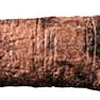#### You may also like### Where Can We Visit?

Charlie and Abi put a counter on 42. They wondered if they could visit all the other numbers on their 1-100 board, moving the counter using just these two operations: x2 and -5. What do you think?### Arithmagons

Can you find the values at the vertices when you know the values on the edges?### Ishango Bone

Can you decode the mysterious markings on this ancient bone tool?

# Babylon Numbers

### Why do this problem ?

This short task gives an interesting historical context and a way to understand the difference between hypothesis and conjecture; convincing argument and proof. It will encourage mathematical creativity and also put students in a situation in which there is no obvious answer or way forward.

Whilst the number skills required are basic, the investigative aspect of the problem would make an interesting challenge for any students who want to develop problem solving skills.

### Possible approach

Discuss the problem. Does anyone have any suggestions? Can others test the suggestions? You will need to use calculators. Students will want to write out the tables and then calculate sums, differences, products and other combinations of the values until they spot a possible pattern.

### Key questions

• Describe what you see in the numbers
• What ways of linking numbers do we know?
• Are there any obvious rows in the table to look at first?
• If there are mistakes in four rows, how many rows do we need to test before rejecting a hypothesis?

### Possible extension

If we find a rule which explains all but the 4 erroneous rows of numbers, does that prove that our rule is the one that the person making the table was thinking of at the time? For what sorts of reasons might a Babylonian have been writing down mathematics? What sort of mathematics might they have needed to know about?

### Possible support

Some students might initially find it difficult to suggest a line of enquiry and then to test it.
Start the group off with this statement 'My hypothesis is that the third column is the sum of the previous two columns' and then ask the class to test out this hypothesis.

For example, is one row the sum of the other two? (No, but it starts a chain of enquiry)